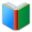# List 1 for 8th Grade MATH

Middle School Words: List 1 for 8th Grade MATH, random words are selected for the Word Quiz worksheet. This sheet is created dynamically, every sheet is different from previous ones. You can download and print the worksheet from browser directly.
 Actions upon current list

 All lists of current grdae
Spelling Words:

Arts Words:

Social Words:

Math Words:

Science Words:

Literature Words:Animal FarmThe Hound of the Baskervilles
 Worksheet - Middle School Words: List 1 for 8th Grade MATH

 Print: List 1 for 8th Grade MATH

Exam Word - https://www.examword.com/

Middle School Words: List 1 for 8th Grade MATH

 1: the set of values of the dependent variable for which a function is defined range of a function[__] lateral area[__] Pythagorean theorem[__] scientific notation[__] 2: a type of display using Cartesian coordinates to display values for two variables for a set of data linear inequality[__] lateral area[__] slope[__] scatter plot[__] 3: invariable; repeating; continually occurring; persistent linear inequality[__] formula[__] domain[__] constant[__] 4: are angles that have a common ray coming out of the vertex going between two other rays, with no overlap of the regions range of a function[__] scientific notation[__] adjacent angles[__] domain[__] 5: a method of writing or displaying numbers in terms of a decimal number between 1 and 10 multiplied by a power of 10 domain[__] constant[__] scientific notation[__] hypotenuse[__]